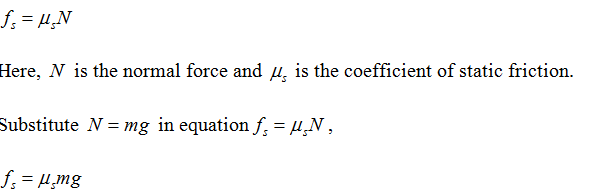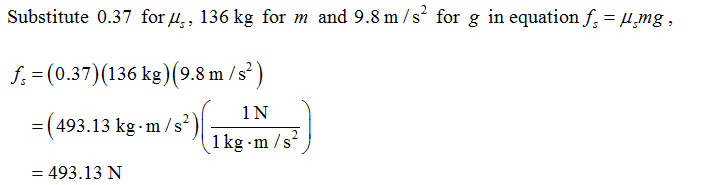Click to Chat

1800-1023-196

+91-120-4616500

CART 0

• 0

MY CART (5)

Use Coupon: CART20 and get 20% off on all online Study Material

ITEM
DETAILS
MRP
DISCOUNT
FINAL PRICE
Total Price: Rs.

There are no items in this cart.
Continue Shopping
```
A 136-kg crate is at rest on the floor. A worker attempts to push it across the floor by applying a 412-N force horizon- tally. (a) Take the coefficient of static friction between the crate and floor to be 0.37 and show that the crate does not move. (b) A second worker helps by pulling up on the crate. What minimum vertical force must this worker apply so that the crate starts to move across the floor? (c) If the second worker applies a horizontal rather than a vertical force, what minimum force, in addition to the original 412-N force, must be exerted to get the crate started?

```
5 years agoNavjyot Kalra
654 Points
```							(a)The magnitude of static force of friction is:Here, m is the mass of the crate, and g is the acceleration due to gravity.Round off to three significant figures,fs = 493 NThe magnitude of applied force by worker is F = 412 N , and is smaller than the static force of friction.Therefore, the crate will not move.(b)Assume that force F1 is applied vertically by another worker, the magnitude of static force of friction would be f’s = μsN’It is important to note that the normal force in this case would be given as:Here m is the mass of the crate, and g is the acceleration due to gravity.The block will start to move when the magnitude of the applied force is equal to the magnitude of static force of friction, that isRound off to three significant figures,F1 = 219 NTherefore, the vertical force applied by the second worker is 219 N .Therefore, a horizontal force of 81 N would be enough to put the crate in motion.
```
5 years ago
Think You Can Provide A Better Answer ?

## Other Related Questions on Mechanics

View all Questions »### Course Features

• 101 Video Lectures
• Revision Notes
• Previous Year Papers
• Mind Map
• Study Planner
• NCERT Solutions
• Discussion Forum
• Test paper with Video Solution### Course Features

• 110 Video Lectures
• Revision Notes
• Test paper with Video Solution
• Mind Map
• Study Planner
• NCERT Solutions
• Discussion Forum
• Previous Year Exam Questions